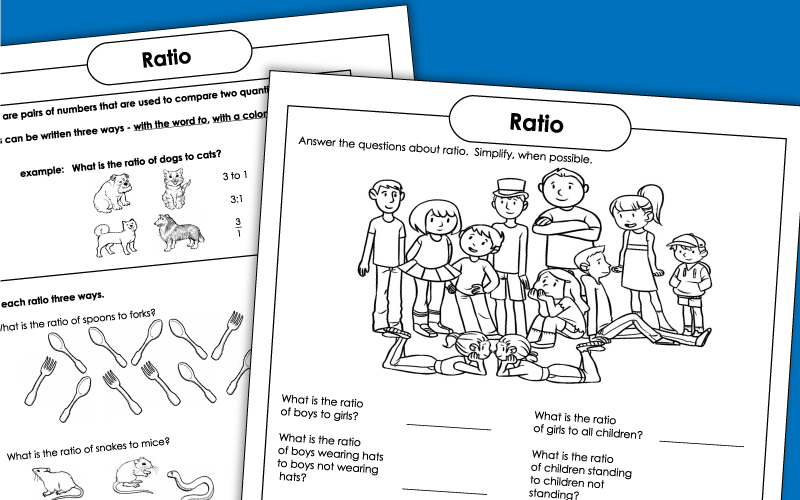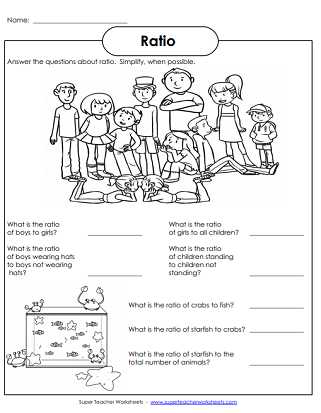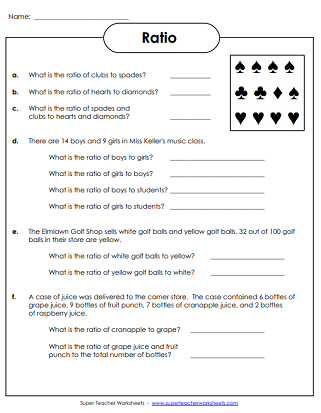# Ratio Worksheets

Compare two quantities with ratios. These worksheets feature basic and intermediate-level ratio activities.Look carefully at each picture and answer the question about the ratio of objects. Write each ratio three different ways.
This worksheet has a picture of dogs, cats, turtles, and birds. Write ratios in the table to compare the number of each animal.
Answer these challenging ratio thinking questions. Recommended for grades 4 and up. Requires some knowledge of percents.
Look at the pictures and answer the questions about the ratios of the objects shown.
Look carefully at each picture and answer the questions about ratio. This worksheet requires students to simplify their answers.
The coins and dollar bills in Taylor's piggy bank are show. Write the ratios of coins and bills.
Probability Worksheets

Learn to calculate the probability of certain events with these printable worksheets.

Math Worksheets

This page contains the full index of math worksheets on our website.

## Images of WorksheetsMy Account
Site Information